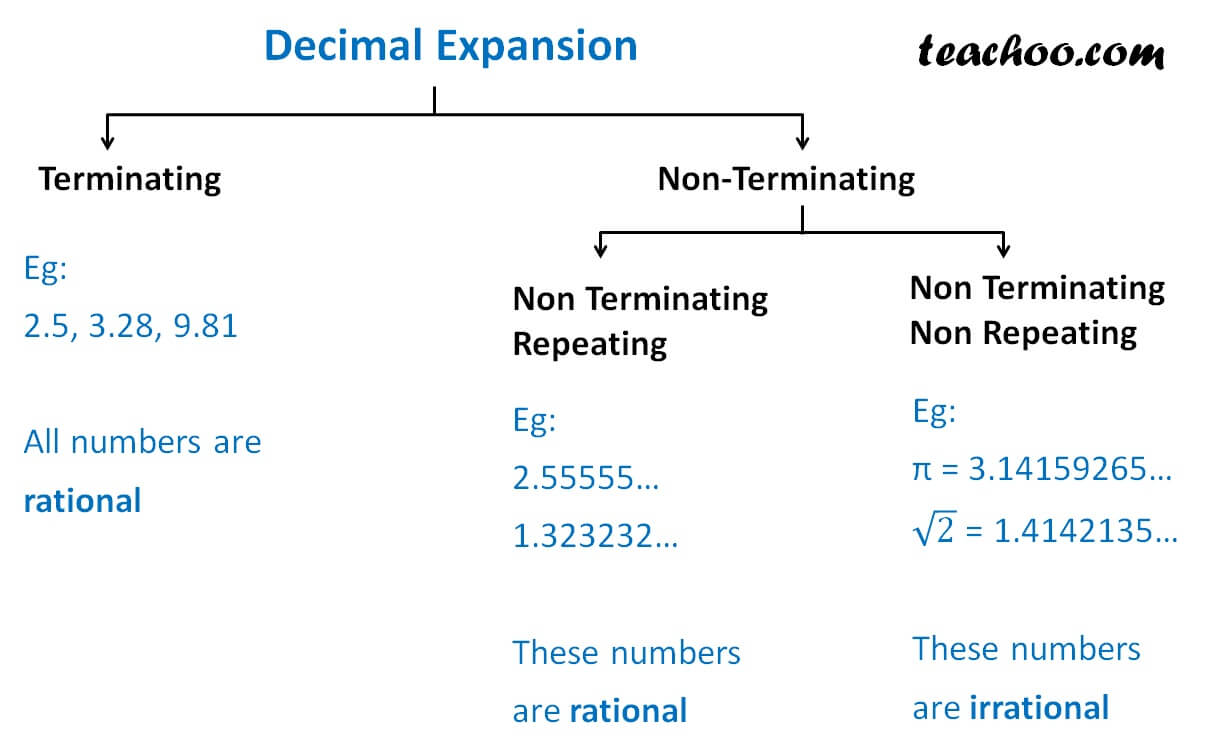Classifiying rational/irrational

Chapter 1 Class 9 Number Systems
Concept wise

Let us find if decimal numbers are rational or irrationalLet us look at some examples

Q: Is 2.5 a rational number?

-a-

Since 2.5 has a terminating expansion

So, it is a rational number.

-ea-

Q: Is 0.142857142… a rational number?

-a-

0.142857142… = 0.1428571428570142857…

Since the terminal expansion is non- terminating repeating.

It is a rational number.

-ea-

Q: Is 1.10100100010000.. a rational number?

-a-

1.10100100010000..

Since the terminal expansion is non- terminating non-repeating.

It is an irrational number.

-ea-

Learn in your speed, with individual attention - Teachoo Maths 1-on-1 Class